﻿ Universe, a Spacetime Harmonic Oscillator

### Universe, a Spacetime Harmonic Oscillator

M. Khoshsima

International Journal of Physics

## Universe, a Spacetime Harmonic Oscillator

Department of Physics, Adelphi University, New York, USA

### Abstract

Energy field waves propagate in the fabric of spacetime. Interaction between spacetime field propagation and matter will generate physical photons. There are three regions of spacetime; (1) events in timelike region corresponding to the expanding universe, (2) events in lightlike region, the fabric of spacetime corresponding to spacetime with no expansion, (3) events in spacelike region corresponding to residual or evanescent universe. Universe is similar to a harmonic oscillator with two phase, right and left expansions. The equilibrium position for a two phase universe is the fabric of spacetime with surge of the stored energy in a singularity, expanding into the next phase of expansion. The evanescent universe is the spacelike event region where mass will decay. Expansion of universe and creation of matter is due to energy field propagation and superposition of energy fields in the fabric of spacetime.

• M. Khoshsima. Universe, a Spacetime Harmonic Oscillator. International Journal of Physics. Vol. 4, No. 1, 2016, pp 21-25. http://pubs.sciepub.com/ijp/4/1/4
• Khoshsima, M.. "Universe, a Spacetime Harmonic Oscillator." International Journal of Physics 4.1 (2016): 21-25.
• Khoshsima, M. (2016). Universe, a Spacetime Harmonic Oscillator. International Journal of Physics, 4(1), 21-25.
• Khoshsima, M.. "Universe, a Spacetime Harmonic Oscillator." International Journal of Physics 4, no. 1 (2016): 21-25.

 Import into BibTeX Import into EndNote Import into RefMan Import into RefWorks

1
Prev Next

### 1. Introduction

Although the energy propagations of sound or water waves require medium of propagation, in the theory of special relativity (SR) light/electromagnetic (EM) waves do not require any medium for propagation in the empty space (vacuum). However, according to the theory of relativity empty space is the four dimensional spacetime in which one can conceptually consider being the medium for the EM wave propagation. That is the reason why a medium such as ether is not required for light propagation. Therefore, it is possible to consider EM propagation being the actual oscillatory propagation of spacetime itself.

One can understand the characteristic of spacetime by analyzing the invariant interval given in the SR. There are three regions of spacetime corresponding to the three events happening in the SR. The “essence” or the “fabric” of spacetime which we refer to as “f-spacetime,” is the actual spacetime prior to expansion. This will correspond to zero invariant interval where the events occur within the non-expanded spacetime. The distinguishing characteristic of this region is not only being the essence and “ground state” of spacetime, it is also the medium which “intrinsic” propagation velocity is equal to that speed of light. F-spacetime is void of space and time.

The timelike event corresponds to the expanding universe or the expanded spacetime where matter can exist. This is our observing universe. The spacelike event, on the other hand will correspond to the “residual” or “evanescent universe.” If matter in timelike region gains a velocity greater than that of speed of light, it instantly will be decaying into evanescent universe by tunneling through the f-spacetime. Mass in the spacelike region will not only be decaying, it also will be imaginary.

Based on the above discussion, universe can considered being a two phase spacetime harmonic oscillator. That is, Universe expands from its equilibrium point which can be identified as f-spacetime to its maximum in the timelike region where it then will collapse to the singularity of f-spacetime with all the stored energy. Afterward it will instantly expand to the next phase opposite to that of the equilibrium where it becomes the next timelike region. The singularity of the universe is the instant moment in which there remain nothing but fabric of spacetime (f-spacetime) and the aggregation of all the stored energy. The stored energy in the singularity will force its way as propagating energy fields causing expansion of f-spacetime. The superposition of energy fields in the f-spacetime will also be the determining factor for the creation of particles and anti-particles in the expanding universe.

### 2. Propagation of Energy Fields

As we know, water is the medium for the propagation of energy in the form of water waves and similarly, air is the medium for the propagation of sound energy. As a consequence of propagation of energy in the form of waves, it was also assumed that light would propagate in a medium known as ether. However, according to the theory of SR light propagates in the empty space and does not require medium of propagation. On the other hand considering vacuum being identical to spacetime, one can assume light or EM waves propagate through the medium in which we can identify as the “fabric” of spacetime (f-spacetime). Spacetime is “everywhere” and such propagation can also happen everywhere in the universe. However, we do make a distinction between f-spacetime (fabric of spacetime with no expansion) and the expanded spacetime which is the present universe, although we refer to both as spacetime. In what follows we shall intent to clarify this. Let us recall invariant interval in Cartesian coordinate system given in special relativity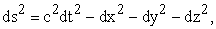(1)

where we have chosen the Minkowski metric component to be of the form (1, -1, -1, -1). Eq. (1) describes the invariant interval between two events. The interval is due to the events that happen in the expanded spacetime (timelike event). In order to reach the fabric of spacetime without expansion (i.e. zero spacetime expansion), one should set the invariant interval ds2 identically equal to zero. This off course will generate the equation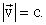(2)

Eq. (2) is an expression for the characteristic velocity of propagation of spacetime that is equal to the speed of light. Light in the form of photon is the quanta of energy. Therefore, light in essence is spacetime energy wave (EM waves) propagation in the f- spacetime where the invariant interval is equal to zero. Quanta of energy or photon, is manifested when the spacetime field wave is scattered by matter. The transfer of momentum of spacetime wave to that of matter is that which generates the photon. Therefore, it is important to note that it is not the photon that propagates in the empty space rather it is the spacetime wave propagation having impact with matter that generates photon. The energy wave (field) propagation therefore, either can occur through matter such as energy of sound waves or through the f-spacetime oscillation.

### 3. Special Theory of Relativity and Boundaries of the Spacetime

The fabric of spacetime where v = c is the “criteria.” We know that every observer in the expanding universe where (ds2 > 0, timelike event), is subject to SR. It happens that as a consequence of SR, speed of light (c) is constant for all such observers. Reciprocally, in the inertia frame of c (f-spacetime), all other observers are at stand still, with zero velocity. One can thereby proclaim that the absolute observer is in the inertia frame of the fabric of spacetime where ds2 = 0. It is this observer that measures spacetime at “stand still.” According to the invariant interval in Eq. (1) there can only be three events, namely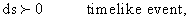(3a)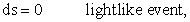(3b)(3c)

We are “realistically” familiar with inequality (3a) and Eq. (3b). As we have mentioned before, Eq. (3b) is the criteria. It is because Eq. (3b) pertains to the actual fabric of spacetime and it is the absolute observer in the sense that it measures rest of the universe at rest. The inequality (3a) describes the events happening in the expanded universe where ds2 is greater than zero. Schematically, this will correspond to an event not in the f-spacetime rather on the “boundary” of it (the expanding universe). In Figure 1. we have illustrated the events corresponding to relations (3a) and (3c) and Eq. (3b) in a flat spacetime.Download asVeiw figureFigures index
Figure 1. Straight line: Lightlike event corresponding to the “fabric” of spacetime where, ds2 =0. Boundary above the straight line: Timelike event corresponding to expanding universe where ds2 >0. Boundary below the straight line: Spacelike event corresponding to evanescent spacetime universe where ds2 <0

We need to understand the inequality (3c) where it corresponds to spacelike events. In special relativity it is a forbidden event where it corresponds to an imaginary spacetime invariant interval. This event is depicted below the fabric of spacetime in Figure 1. where it pertains to (ds2 <0) and can be explained by recalling the relativistic mass(4a)

where γ is the relativistic constant. In the region ds2 > 0, the expanding universe, mass is positive real and increase in magnitude with velocity v, as long as it is not equal or greater than c. At the fabric of spacetime where it has the characteristic of field propagation of v = c, a mass travelling with speed of light becomes infinite and therefore a singularity in spacetime. In other words, if mass gains a velocity of that speed of light it automatically inhibits the characteristic of f-spacetime where no massive particle are allowed. This region only allows energy wave propagation that eventually may manifest as particles. It is also interesting to note that according to the Eq. (4a) if mass acquires a velocity greater than speed of light it (mass) becomes imaginary and begins to decay with increasing velocity. The spacelike region where mass becomes imaginary is the analog of materials with imaginary propagation or dielectric constants where electromagnetic fields decay as evanescent waves(4b)

where α > 1 is constant. In Figure 2. we have plotted relativistic mass versus velocity. Notice the particle at rest with mass m0 in ds2 > 0 region, an event in timelike expanding universe. As m0 begins to move, its relativistic mass also begins to increase. There will be a pole (singularity) at v = c where mass cannot be tolerated in timelike event region. C is a property of fabric of spacetime only, velocity of intrinsic field oscillation. A massive particle with v = c cannot also be tolerated in lightlike event region. A particle therefore cannot have v = c anywhere in spacetime; It will be singularity in both f-spacetime and the expanding universe as shown in Fig. 2. It however, can leap through singularity and gain higher velocity than c into the evanescent region. Once the massive particle approaches speed v > c, it then will tunnel through the ds2 = 0 region, into the ds2 < 0 [Fig. 1.], where it will decay as shown in Figure 2.Download asVeiw figureFigures index
Figure 2. Relativistic mass vs. Velocity

### 4. Two Phase Universe, a harmonic Oscillator

The region in which we have identified as fabric of spacetime is simply spacetime in its essence, void of space and time (spacetime with no expansion). As an example consider a volume of mass-less little strings connected to one another while being at equilibrium. Let then apply an internal pulling force to the little strings which will cause their elongation. This would correspond to f-spacetime at equilibrium and the corresponding expanding universe. The difference however is that in reality f-spacetime contrary to the little strings is a conceptual object with zero mass and zero volume. The f-spacetime is therefore, a zero spacetime.

The internal energy does have a decisive rule in the expansion of spacetime and the creation of the particles. Consider a harmonic oscillator where due to its initial potential energy it will oscillate about its equilibrium position. The spring will expand from equilibrium to its maximum extension, it then contracts and expands to the other side of the equilibrium. Universe also due to its intrinsic potential energy behaves in the same manner. Universe by analogy to a harmonic oscillator has two major “components.”The fabric of spacetime, analog to the mass-less string and the potential energy driving this fabric in the form of kinetic energy and expansion. The internal kinetic energy of the universe is independent from the fabric of spacetime. This energy not only is the cause for the accelerated expansion of the universe (similar to a harmonic oscillator), it also is responsible for the creation of matter; after all matter can consider being a “solidified” form of energy. As we know energy and matter are intrinsically related through the speed of light c, which we now know it is the characteristic propagation of f-spacetime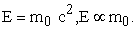(5)

There are two phases of universe in which both phase will include the expansion and the evanescent region, i.e. ds2 > 0 and ds2 < 0 as well as fabric of spacetime ds2 = 0. We will refer to these two phases as “right and left expansions.” If we assume currently being at the right expansion then the right expansion will possess timelike events and consequently the left will be the residual or spacelike region, namely(6a)

and if be on the left expansion,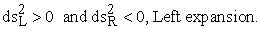(6b)

An example of two phase expansion of universe can be two empty balloons attached at the very ends. This corresponds to the fabric of spacetime empty of energy with no spacetime expansion. Now assume the right balloon being instantaneously bloated by air to its maximum. Then the air from the right balloon will enter to the left through the neck end. In this example there will be an equilibrium state where both balloons will be partially expanded. In two phase universe theory however, we have to assume one side completely being expanded and then complete contraction to the singularity into f-spacetime (ds2 = 0) with then expansion into the other side. This requires a universe with initial condition similar to that of an ideal LC (inductor and capacitor) circuit where oscillation will be indefinite. From our point of view all that we observe is the universe expansion and contraction to singularity and the beginning of a new big bang. The right and left expansions become irrelevant.

### 5. Creation of Matter and Photon

The energy stored in the singularity (ds2 = 0 region) after complete contraction of the universe will then cause the expansion of spacetime into second phase. As we have indicated, energy in the universe either propagates through the fabric of spacetime in the form of spacetime energy fields or propagation through matter. The energy propagations through matter are trivial. The energy propagation through spacetime will physically manifest only when spacetime vibrations having momentum impact with matter or through the superposition of the f-spacetime fields.

At the moment of big bang there will be tremendous amount of energy propagating in ds2 = 0 that will lead to the expansion of fabric of spacetime. Similar to the expansion of matter (i.e. substance or gas) that is caused by the energy fields transferring kinetic energy to atoms and particles, energy field fields will also transfer kinetic energy to the f-spacetime in the form of expansion.

The propagating energy field waves in the ds2 = 0 region will also interact with one another which will lead to generation of particles and anti-particles in the ds2 > 0. This cannot happen prior to the expansion of spacetime into timelike region. In other words, creation of particles and anti-particles in the universe are the result of the f-spacetime energy waves’ superposition while universe began expanding. Reciprocally, once matter and antimatter occupy same event in the ds2 > 0 region, they immediately will annihilate into f-spacetime energy field waves. It is not the energy however, that causes the interaction, it rather is the transfer of relativistic momentum as shown in Eq. (7)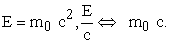(7)

Photons are therefore created in two ways, either through interaction of f-spacetime wave fields with matter in the form of momentum exchange or matter and antimatter annihilations. In any event, photon as a physical object will manifest only when f-spacetime energy waves interact as momentum exchange to that of matter. Matter interactions in the particle level are such that it may generate new particles that are particular superposition of spacetime waves with some additional energy that will appear in f-spacetime. Additional energies remain until being manifested in the form of photons, or through superposition will later manifest as other forms of massive particles. We noticeably realize that particles are energy manifestation of lightlike or f-spacetime vibrations into a timelike spacetime. The contraction of universe to a singularity will eventually bring all particles and antiparticles into the same exact point of spacetime event that will lead to their annihilation into f-spacetime energy field waves. In Figure 3(a) and Figure 3(b) we have depicted the creation of particle and anti-particle through superposition into the expanding universe and decay of mass into evanescent spacetime for both right and left expansions.Download asVeiw figureFigures index
Figure 3. (a) Right expansion: superposition of energy waves creating particles and anti-particles in the expanding universe on the right, with imaginary matter decaying in the evanescent universe on the left. (b) Left expansion: superposition of energy waves creating particles and anti-particles in the expanding universe on the left, with imaginary matter decaying in the evanescent universe on the right

### 6. Quantum States of Matter and Anti-matter

Figure 3 shows the schematic for creation of particle and anti-particle pertain to the right and left expansions. The superposition of energy spacetime field waves in ds2 = 0 will lead to the generation of particles and anti-particles into the expanded universe. This process can also happen in opposite directions. That is to say, matter and anti-matter may annihilate into propagation in f-spacetime. We can also expect the tunneling of matter through f-spacetime into the evanescent region. In this region as particle’s velocity approaches infinity, the mass will decay to zero. It happens that once particle’s speed reduces to v < c, according to Eq. (4a) the particle will no longer exist in the evanescent region, rather will tunnel back to ds2 > 0 region and reappear once again in the expanding universe.

There are only a limited permitted amount of energy wave superposition that can leap through the interface and appear as types of particles and anti-particles which universe contains. Once particle appears in the timelike event, its mass will correspond to the half of the total energy of the superposition field waves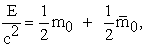(8)

where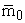is the mass of anti-particle. That which distinguishes a particle from its anti-particle partner is the charge it acquires. The anti-particle of a particle with charge q will have a charge of (-q). Namely,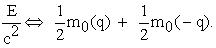(9)

The Left hand side of Eq. (9) represents the superposition of energy waves in the f-spacetime. The right hand side of Eq. (9) expresses the creation of particle and anti-particle. If we assign a charge eigen-state to a massive particle, let say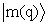, then the overlap of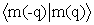should lead to the annihilation of the masses and the release of energy in the form of f-spacetime field waves as is expressed in Eq. (9). A massive particle cannot be without a charge quantum state. A neutral particle such as neutron for example, is such that the superposition of total charge eigen-values within that particle is zero. A particle and anti-particle will come into existence (manifestation of energy in the form of matter in ds2 > 0 region) in the general form of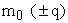with also having other distinguished quantum numbers. However, it is the charge quantum number that will facilitate particle/anti-particle annihilation into f-energy field waves. The two universal attribute of particles pertain to spacetime discussion are therefore, rest mass and charge. Particle and anti-particle states in the form of matter will shatter into energy, once charge quantum states of the two interacting particle-anti-particle are vanished. We can therefore say charge is the “glue” that which localizes energy in the form of matter and if vanish, the energy no longer can be localized in timelike spacetime event in the form of matter, rather it will be energy propagating in lightlike f-spacetime. We should notice that in timelike spacetime energy is localized where as in lightlike spacetime energy is propagative.

Fundamental particles are the least number of possible superposition in f-spacetime fields whereas the composite particles (non-fundamental) are the consequence of additional superposition. Mass is the localized superposition of energy field states in the expanding spacetime. The localized field superposition carry charge quantum states that are responsible for localization of the energy fields in the form we know as matter.

### 7. Conclusion

Our universe is comprised of three events in special relativity i.e., timelike, lightlike and spacelike which correspond to the three regions of spacetime. The timelike region is the present expanding universe in which events are real and mass cannot acquire a speed equal or greater than speed of light. The lightlike region corresponds to the fabric of spacetime where its essential form is the universe prior to expansion and when it contains all the intrinsic potential energy, it will manifest instantly as big bang in a singularity. The spacelike region corresponds to the evanescent spacetime or the residual universe. It is the region where masses will manifest, however, if acquire a speed greater than speed of light and will be subject to decay.

The three regions of spacetime associated with expansion, singularity and evanescence will prompt us to come to the logical conclusion that universe has a two phase or, the right and left expansions. This is indeed similar to a harmonic oscillator where each phase is the symmetric analog of other.

The fabric of spacetime is the medium in which all energy fields are contained while propagating with the characteristic speed, c. In the instant of the big bang the stored energy (propagating fields) in the lightlike region will flow in the form of expansion of fabric of spacetime. The fabric of spacetime in itself is physically void of space and time, however, instant surge of flow of super kinetic energy from the contraction of let say right universe will cause the expansion into next phase (left expansion). Energy can only be stored in the form of propagating fields that will cause the expansion of spacetime whereas their superposition will cause particle and anti-particle creation. In other words the propagation of energy fields will cause the expansion and their superposition will cause the creation of matter and anti-matter in the spacetime.

### References

  D. Simpson, “A Mathematical Derivation of the General Relativistic Schwarzschild Metric,” 2007In article  S. Hawking, “A Brief History of Time,” New York: Bantam Books, 1988.In article PubMed  R.L. Faber, “Differential Geometry and Relativity Theory,”: An Introduction. New York: Marcel Dekker, Inc., 1983.In article  T. Marsh, “General Relativity,” class notes, 2009In article  “S. Chandrasekhar, “The Mathematical Theory of Black Holes”. Clarendon Press (1983)In article  C.W. Misner, K.S. Thorne, and J.A. Wheeler, “Gravitation”. Freeman (1973).In article  S. Weinberg, “Gravitation and Cosmology: Principles and Applications of the General Theory of Relativity”. Wiley (1972)In article  G. ‘t Hooft, “ Introduction To General Relativity,” Institute for Theoretical Physics; Utrecht University (2002)In article  Poul Olesen, “General Relativity and Cosmology,” The Niels Bohr Institute (2008).In article  M. Kachelrieb, “Gravitation and Cosmology,” Institute for fysikk; NTNU (2010).In article  M. Khoshsima, “Black Hole Spacetime Equation in Special Relativity.” International Journal of Astronomy, Astrophysics and Space Science; Vol. 2, No. 4, 2015, pp. 30-33.In article